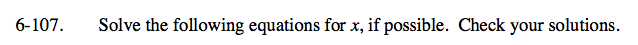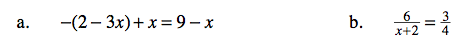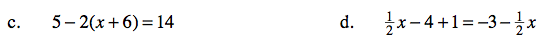### Home > CAAC > Chapter 6 > Lesson 6.3.1 > Problem6-107

6-107.
1. Solve the following equations for x, if possible. Check your solutions. Homework Help ✎

1. −(2 − 3x) + x = 9 − x

2.3. 5 − 2(x + 6) = 14

4.− 4 + 1 = −3 −To solve for x you need to isolate the variable, or get it alone.

Distribute
−2 + 3x + x = 9 − x
4x − 2 = 9 − x
Isolate variable by adding 2 and an x to both sides.
5x = 11
Divide
x = 2.2